# clustra: clustering trajectories

#### 2022-01-16

The clustra package was built to cluster longitudinal trajectories (time series) on a common time axis. For example, a number of individuals are started on a specific drug regimen and their blood pressure data is collected for a varying amount of time before and after the start of the medication. Observations can be unequally spaced, unequal length, and only partially overlapping.

Clustering proceeds by an EM algorithm that iterates switching between fitting a bspline to combined responses within each cluster (M-step) and reassigning cluster membership based on nearest fitted bspline (E-step). Initial cluster assignments are random. The fitting is done with the mgcv package function bam, which scales well to very large data sets.

For this vignette, we begin by generating a data set with the gen_traj_data() function. Given its parameters, the function generates groups of ids (their size given by the vector n_id) and for each id, a random number of observations based on the Poisson($$\lambda =$$ m_obs + 3) distribution. The 3 additional observations are to guarantee one before intervention at time start, one at the intervention time 0, and one after the intervention at time end. The start time is Uniform(s_range) and the end time is Uniform(e_range). The remaining times are at times Uniform(start, end). The time units are arbitrary and depend on your application. Up to 3 groups are implemented so far, with Sin, Sigmoid, and constant forms.

We also set RNGkind and seed for reproducibility. Code below generates the data and looks at a few observations of the generated data. The mc variable sets core use and will be assigned to mccores parameter through the rest of the vignette. By default, 1 core is assigned. Parallel sections are implemented with parallel::mclappy(), so on unix and Mac platforms it is recommended to use the full number of cores available for faster performance.

library(clustra)
mc = 1 # If running on a unix or a Mac platform, increase up to 2x # cores
set.seed(12345)
data = gen_traj_data(n_id = c(400, 800, 1600), m_obs = 25,
s_range = c(-365, -14), e_range = c(0.5*365, 2*365),
noise = c(0, 5))
head(data)
##      id time  response true_group
## 1: 4626 -112  90.40934          2
## 2: 4626 -112  88.24385          2
## 3: 4626 -104  87.74245          2
## 4: 4626  -71  96.42432          2
## 5: 4626  -15 108.24412          2
## 6: 4626   -9 100.72032          2

Select a few random ids and print their scatterplots.

library(ggplot2)
ggplot(data[id %in% sample(unique(data[, id]), 9)],
aes(x = time, y = response)) + facet_wrap(~ id) + geom_point()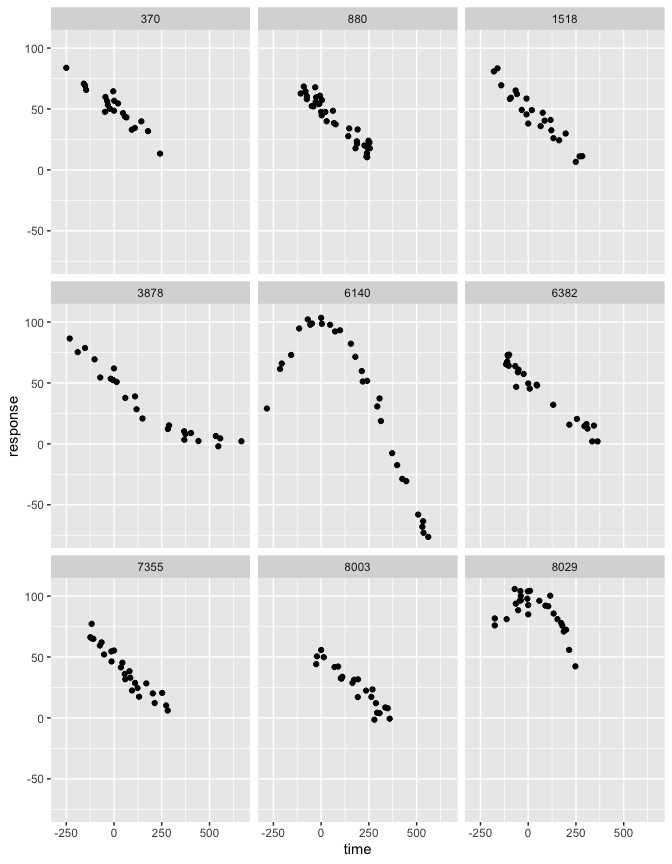Next, cluster the trajectories. Set k=3, spline max degrees of freedom to 30, and set conv maximum iterations to 10 and convergence when 0 changes occur. mccores sets the number of cores to use in various components of the code. Note that this does not work on Windows operating systems, where it should be left at 1. In the code that follows, we use verbose output to get information from each iteration.

set.seed(1234737)
cl = clustra(data, k = 3, maxdf = 30, conv = c(10, 0), mccores = mc,
verbose = TRUE)
##
##  1 (M-step 123)1.1 (E-step 12345)0.4 Changes: 1816 Counts: 575 480 1745 Deviance: 74804226
##  2 (M-step 123)0.3 (E-step 12345)0.5 Changes: 507 Counts: 378 674 1748 Deviance: 41658264
##  3 (M-step 123)0.4 (E-step 12345)0.3 Changes: 386 Counts: 402 756 1642 Deviance: 19700646
##  4 (M-step 123)0.3 (E-step 12345)0.3 Changes: 29 Counts: 404 775 1621 Deviance: 4366500
##  5 (M-step 123)0.3 (E-step 12345)0.3 Changes: 0 Counts: 404 775 1621 Deviance: 1960868
##  Total time: 4.3  converged

Next, plot the raw data (sample if more than 10,000 points). Then repeat the plot with resulting spline fit, colored by the cluster value.

sdt = data
if(nrow(data) > 10000)
sdt = data[, group:=factor(..cl$data_group)][sample(nrow(data), 10000)] ggplot(sdt, aes(x = time, y = response)) + geom_point(pch = ".")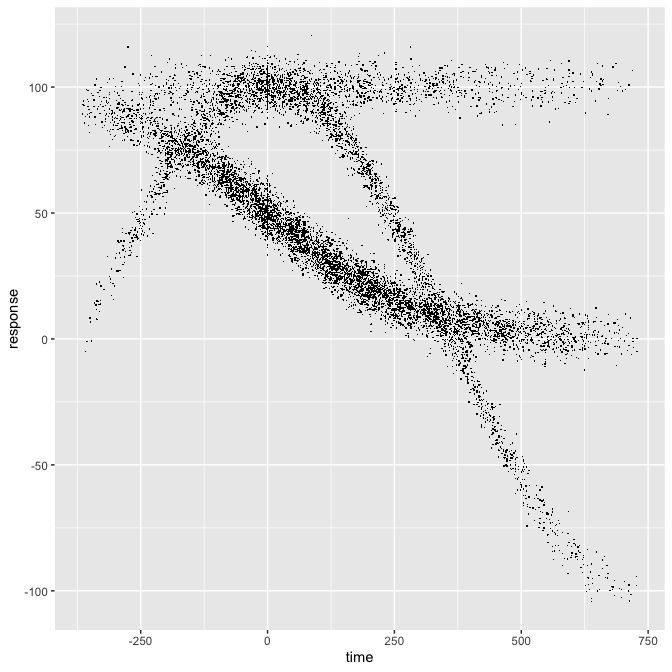np = 100 k = length(cl$tps)
ntime = seq(data[, min(time)], data[, max(time)], length.out = np)
pdata = expand.grid(time = ntime, group = factor(1:k))
pdata = subset(pdata, group %in% which(lengths(cl$tps) > 0)) pred = vector("list", k) for(i in 1:k) if(is.null(cl$tps[[i]])) {
pred[[i]] = NULL
} else {
pred[[i]] = mgcv::predict.bam(cl$tps[[i]], newdata = list(time = ntime), type = "response") } pdata$pred = do.call(c, pred)
ggplot(pdata, aes(x = time, y = pred, color = group)) +
geom_point(data = sdt, aes(y = response), pch = ".") + geom_line()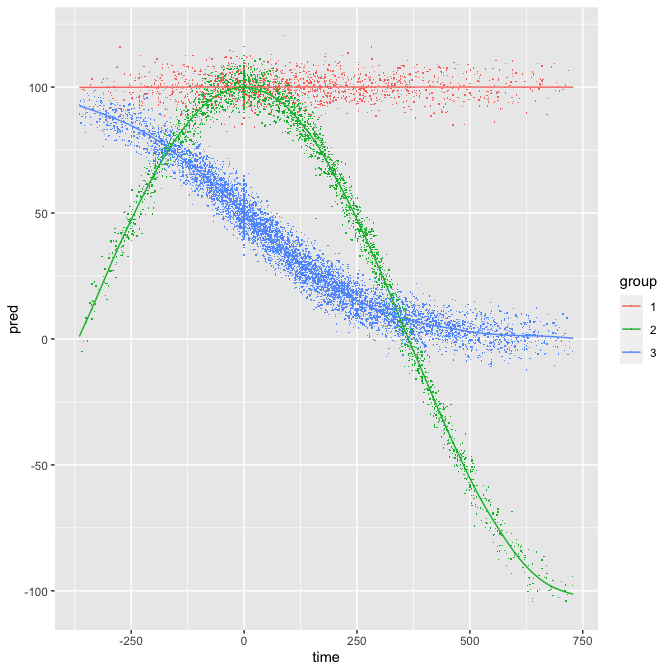The Rand index for comparing with true_groups is

MixSim::RandIndex(cl$data_group, data[, true_group]) ##$R
##  1
##
## $AR ##  1 ## ##$F
##  1
##
## $M ##  0 A perfect score! Let’s double the error variance (4*sd) in data generation … set.seed(1234567) data2 = gen_traj_data(n_id = c(500, 1000, 2000), m_obs = 25, s_range = c(-365, -14), e_range = c(60, 2*365), noise = c(0, 20)) iplot = sample(unique(data2$id), 9)
sampobs = match(data2$id, iplot, nomatch = 0) > 0 ggplot(data2[sampobs], aes(x = time, y = response)) + facet_wrap(~ id) + geom_point()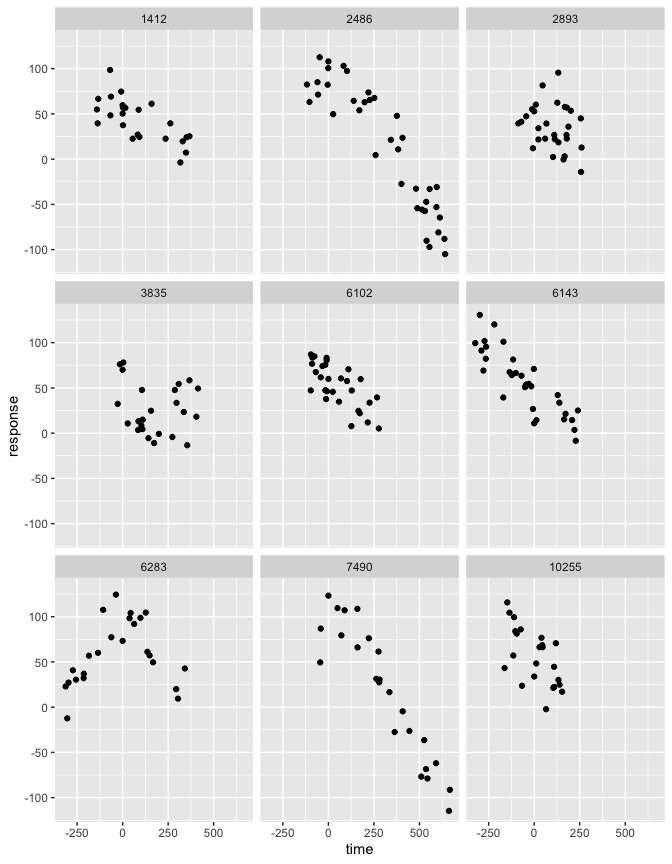cl = clustra(data2, k = 3, maxdf = 30, conv = c(10, 0), mccores = mc, verbose = TRUE) ## ## 1 (M-step 123)0.4 (E-step 12345)0.4 Changes: 2287 Counts: 321 2001 1178 Deviance: 122665878 ## 2 (M-step 123)0.4 (E-step 12345)0.4 Changes: 212 Counts: 487 2013 1000 Deviance: 44760360 ## 3 (M-step 123)0.4 (E-step 12345)0.4 Changes: 24 Counts: 497 2013 990 Deviance: 39425540 ## 4 (M-step 123)0.4 (E-step 12345)0.4 Changes: 4 Counts: 495 2013 992 Deviance: 39411328 ## 5 (M-step 123)0.4 (E-step 12345)0.4 Changes: 0 Counts: 495 2013 992 Deviance: 39411053 ## Total time: 3.9 converged MixSim::RandIndex(cl$data_group, data2[, true_group])
## $R ##  0.994522 ## ##$AR
##  0.9888344
##
## $F ##  0.9936525 ## ##$M
##  52727286

The result is less perfect but still pretty good score. Now the plots:

sdt = data2
if(nrow(data) > 10000)
sdt = data2[, group:=factor(..cl$data_group)][sample(nrow(data), 10000)] ggplot(sdt, aes(x = time, y = response)) + geom_point(pch = ".")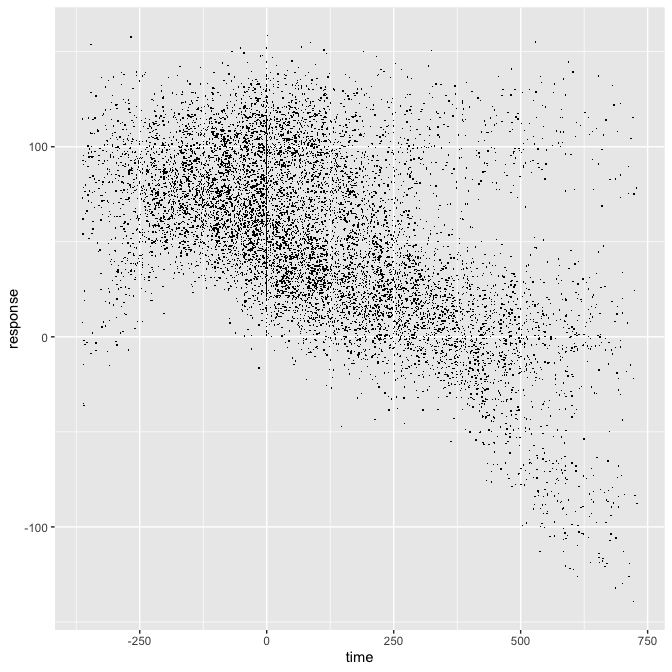np = 100 k = length(cl$tps)
ntime = seq(data[, min(time)], data[, max(time)], length.out = np)
pdata = expand.grid(time = ntime, group = factor(1:k))
pdata = subset(pdata, group %in% which(lengths(cl$tps) > 0)) pred = vector("list", k) for(i in 1:k) if(is.null(cl$tps[[i]])) {
pred[[i]] = NULL
} else {
pred[[i]] = mgcv::predict.bam(cl$tps[[i]], newdata = list(time = ntime), type = "response") } pdata$pred = do.call(c, pred)
ggplot(pdata, aes(x = time, y = pred, color = group)) +
geom_point(data = sdt, aes(y = response), pch = ".") + geom_line()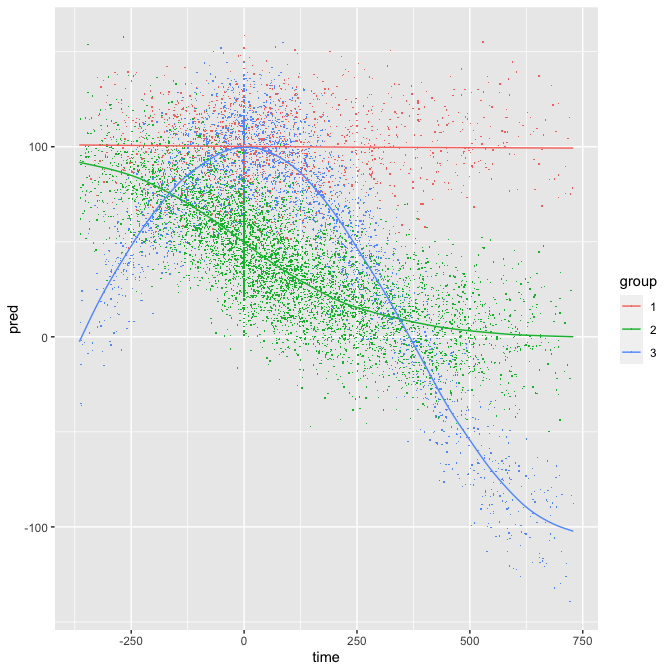Average silhouette value is a way to select the number of clusters and a silhouette plot provides a way for a deeper evaluation (Rouseeuw 1986). As silhouette requires distances between individual trajectories, this is not possible due to unequal trajectory sampling without fitting a separate model for each id. As a proxy for distance between points, we use trajectory distances to cluster mean spline trajectories in the clustra_sil() function. The structure returned from the clustra() function contains the matrix loss, which has all the information needed to construct these proxy silhouette plots. The function clustra_sil() performs clustering for a number of k values and outputs information for the silhouette plot that is displayed next. We relax the convergence criterion in conv to 1 % of changes (instead of 0 used earlier). We use the first data set with noise = c(0, 5).

set.seed(1234737)
sil = clustra_sil(data, k = c(2, 3, 4), mccores = mc, conv = c(7, 1),
verbose = TRUE)
##
##  1 (M-step 123)0.3 (E-step 12345)0.3 Changes: 1348 Counts: 1923 877 Deviance: 75065158
##  2 (M-step 123)0.3 (E-step 12345)0.3 Changes: 106 Counts: 2017 783 Deviance: 37301553
##  3 (M-step 123)0.3 (E-step 12345)0.3 Changes: 57 Counts: 2074 726 Deviance: 30613578
##  4 (M-step 123)0.3 (E-step 12345)0.3 Changes: 30 Counts: 2104 696 Deviance: 29782478
##  5 (M-step 123)0.3 (E-step 12345)0.3 Changes: 21 Counts: 2125 675 Deviance: 29507877
##  Total time: 2.9  converged
##
##  1 (M-step 123)0.3 (E-step 12345)0.3 Changes: 1790 Counts: 815 1606 379 Deviance: 74791223
##  2 (M-step 123)0.4 (E-step 12345)0.3 Changes: 67 Counts: 775 1621 404 Deviance: 5594586
##  3 (M-step 123)0.4 (E-step 12345)0.4 Changes: 0 Counts: 775 1621 404 Deviance: 1960868
##  Total time: 2.1  converged
##
##  1 (M-step 123)0.4 (E-step 12345)0.5 Changes: 2055 Counts: 424 408 451 1517 Deviance: 74939820
##  2 (M-step 123)0.4 (E-step 12345)0.4 Changes: 513 Counts: 152 254 773 1621 Deviance: 20437897
##  3 (M-step 123)0.4 (E-step 12345)0.4 Changes: 181 Counts: 185 219 775 1621 Deviance: 1967647
##  4 (M-step 123)0.4 (E-step 12345)0.4 Changes: 12 Counts: 187 217 775 1621 Deviance: 1953786
##  Total time: 3.3  converged
plot_sil = function(x) {
msil = round(mean(x$silhouette), 2) ggplot(x, aes(id, silhouette, color = cluster, fill = cluster)) + geom_col() + ggtitle(paste("Average Width:", msil)) + scale_x_discrete(breaks = NULL) + scale_y_continuous("Silhouette Width") + geom_hline(yintercept = msil, linetype = "dashed", color = "red") } lapply(sil, plot_sil) ## []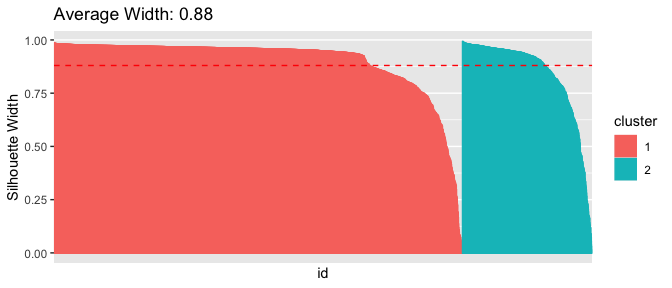## ## []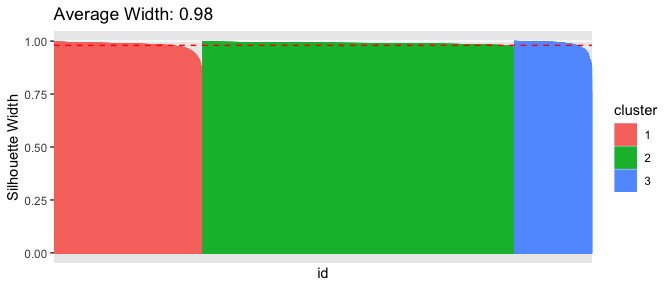## ## []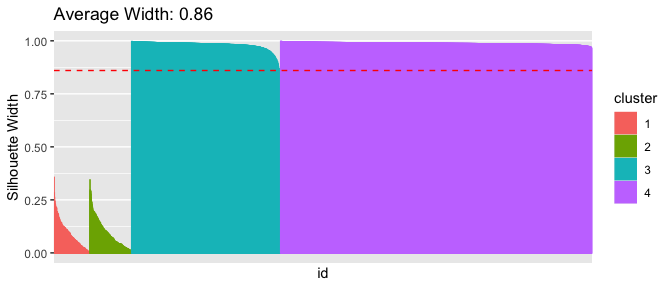The plots show that 3 clusters give the best Average Width. If we don’t want to recluster the data again, we can directly reuse a previous clustra run and produce a silhouette plot for it, as we now do for the double variance error data clustra run above results in cl. sil = clustra_sil(cl) lapply(sil, plot_sil) ## []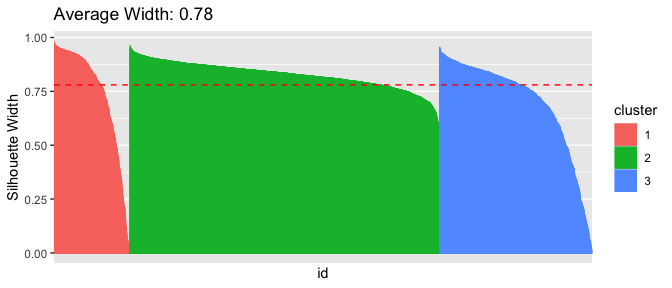Another way to select the number of clusters is the Rand Index comparing different random starts and different numbers of clusters. When we replicate clustering with different random seeds, the “replicability” is an indicator of how stable the results are for a given k, the number of clusters. For this demonstration, we look at k = c(2, 3, 4), and 10 replicates for each k. set.seed(1234737) ran = clustra_rand(data, k = c(2, 3, 4), mccores = mc, replicates = 10, conv = c(7, 1), verbose = TRUE) ## 2 1 iters = 5 deviance = 29507877 xit = converged counts = 2125 675 changes = 21 ## 2 2 iters = 5 deviance = 36249163 xit = converged counts = 1778 1022 changes = 19 ## 2 3 iters = 7 deviance = 29766251 xit = max-iter counts = 2104 696 changes = 29 ## 2 4 iters = 3 deviance = 36066106 xit = converged counts = 1749 1051 changes = 11 ## 2 5 iters = 2 deviance = 37852307 xit = converged counts = 1678 1122 changes = 19 ## 2 6 iters = 3 deviance = 36416817 xit = converged counts = 1017 1783 changes = 24 ## 2 7 iters = 5 deviance = 29579485 xit = converged counts = 683 2117 changes = 23 ## 2 8 iters = 5 deviance = 29548364 xit = converged counts = 681 2119 changes = 22 ## 2 9 iters = 5 deviance = 29587537 xit = converged counts = 2116 684 changes = 23 ## 2 10 iters = 6 deviance = 29579485 xit = converged counts = 683 2117 changes = 23 ## 3 1 iters = 4 deviance = 1960868 xit = converged counts = 775 404 1621 changes = 0 ## 3 2 iters = 4 deviance = 1960868 xit = converged counts = 775 1621 404 changes = 0 ## 3 3 iters = 6 deviance = 1960868 xit = converged counts = 404 775 1621 changes = 0 ## 3 4 iters = 3 deviance = 1994427 xit = converged counts = 404 775 1621 changes = 8 ## 3 5 iters = 3 deviance = 1960868 xit = converged counts = 404 1621 775 changes = 0 ## 3 6 iters = 3 deviance = 2113138 xit = converged counts = 404 1621 775 changes = 21 ## 3 7 iters = 3 deviance = 2101342 xit = converged counts = 775 404 1621 changes = 21 ## 3 8 iters = 3 deviance = 1960868 xit = converged counts = 775 1621 404 changes = 0 ## 3 9 iters = 4 deviance = 1960868 xit = converged counts = 1621 404 775 changes = 0 ## 3 10 iters = 6 deviance = 1960868 xit = converged counts = 404 775 1621 changes = 0 ## 4 1 iters = 4 deviance = 1960868 xit = zerocluster converged counts = 775 1621 404 changes = 0 ## 4 2 iters = 4 deviance = 1960868 xit = zerocluster converged counts = 1621 775 404 changes = 0 ## 4 3 iters = 7 deviance = 1949200 xit = max-iter counts = 404 255 1621 520 changes = 32 ## 4 4 iters = 6 deviance = 1950132 xit = converged counts = 234 1621 404 541 changes = 20 ## 4 5 iters = 7 deviance = 1938540 xit = max-iter counts = 775 1101 520 404 changes = 96 ## 4 6 iters = 7 deviance = 1949601 xit = max-iter counts = 404 519 256 1621 changes = 42 ## 4 7 iters = 4 deviance = 1960868 xit = zerocluster converged counts = 775 404 1621 changes = 0 ## 4 8 iters = 4 deviance = 1960868 xit = zerocluster converged counts = 404 775 1621 changes = 0 ## 4 9 iters = 7 deviance = 1932429 xit = converged max-iter counts = 795 775 404 826 changes = 16 ## 4 10 iters = 7 deviance = 1947034 xit = max-iter counts = 1621 404 504 271 changes = 31 rand_plot(ran)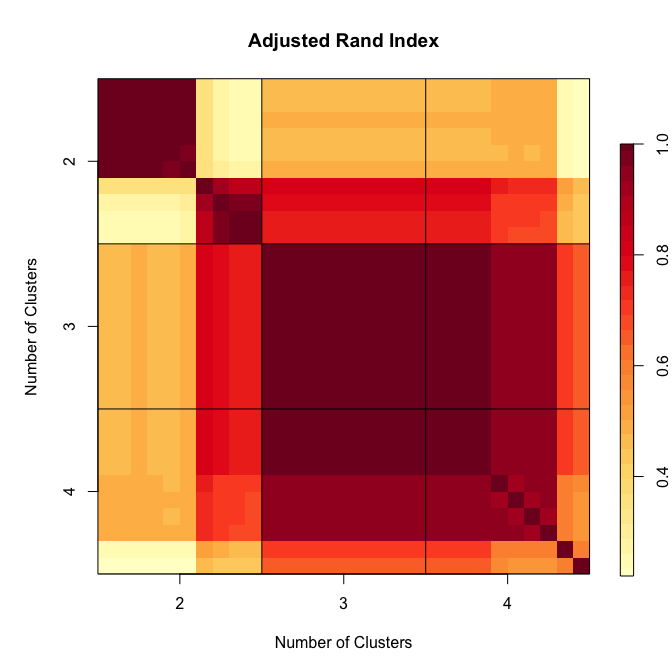The plot shows Adjusted Rand Index similarity level between all pairs of 30 clusterings (10 random starts for each of 2, 3, and 4 clusters). The ten random starts agree the most for k=3. From the deviance results shown during iterations, we also see that all of the k=3 clusters are near the best deviance attainable even with k = 4. Among the k = 4 results, several converged to only three clusters that agree with k=3 results. Another possible evaluation of the number of clusters is to first ask clustra for a large number of clusters, evaluate the cluster centers on a common set of time points, and feed the resulting matrix to a hierarchical clustering function. Below, we ask for 40 clusters on the data2 data set but actually get back only 26 because several become empty or too small for maxdf. Below, the hclust() function clusters the 26 resulting cluster means, each evaluated on 100 time points. set.seed(12347) cl = clustra(data2, k = 40, maxdf = 30, conv = c(10, 0), mccores = mc, verbose = TRUE) ## ## 1 (M-step 123)2.2 (E-step 12345)3.3 Changes: 3375 Counts: 8 69 0 0 0 10 1346 0 0 1083 60 21 2 45 11 0 4 0 19 0 0 8 6 0 3 197 86 6 0 388 11 0 34 1 8 49 0 6 0 19 Deviance: 119895337 ## 2 (M-step 123)0.9 (E-step 12345)1.7 Changes: 3344 Counts: 47 90 75 456 936 31 52 33 108 49 32 150 39 139 48 23 162 93 387 24 104 85 124 25 86 102 Deviance: 39952143 ## 3 (M-step 123)1.4 (E-step 12345)2.1 Changes: 699 Counts: 86 114 102 352 841 49 86 32 101 121 35 136 57 163 55 47 154 119 291 28 138 68 136 39 81 69 Deviance: 37284663 ## 4 (M-step 123)1.4 (E-step 12345)2.1 Changes: 496 Counts: 99 134 118 277 747 53 106 28 92 216 34 136 58 178 63 55 158 117 242 39 147 72 138 40 100 53 Deviance: 36918096 ## 5 (M-step 123)1.4 (E-step 12345)2.2 Changes: 404 Counts: 111 153 131 234 674 51 114 28 86 281 43 135 65 178 71 51 161 131 196 40 165 68 134 44 99 56 Deviance: 36703348 ## 6 (M-step 123)1.4 (E-step 12345)2.1 Changes: 321 Counts: 126 153 140 205 604 52 125 27 83 332 46 144 65 172 78 53 157 129 178 43 172 68 130 60 104 54 Deviance: 36526685 ## 7 (M-step 123)1.4 (E-step 12345)2.1 Changes: 260 Counts: 133 155 152 188 555 52 142 27 80 358 45 145 67 169 88 53 152 125 161 46 175 68 119 82 109 54 Deviance: 36390623 ## 8 (M-step 123)1.4 (E-step 12345)2.1 Changes: 185 Counts: 139 146 163 176 528 53 147 28 79 371 44 149 68 165 98 54 144 134 155 46 177 67 113 96 107 53 Deviance: 36287317 ## 9 (M-step 123)1.4 (E-step 12345)2.2 Changes: 140 Counts: 141 140 164 166 509 53 145 30 78 377 46 154 68 165 105 54 144 132 153 46 177 65 110 108 117 53 Deviance: 36223382 ## 10 (M-step 123)1.5 (E-step 12345)2.2 Changes: 133 Counts: 141 135 167 162 489 53 152 30 78 385 49 159 63 162 104 55 143 134 151 49 176 65 108 116 119 55 Deviance: 36179515 ## Total time: 36.5 zerocluster max-iter gpred = function(tps, newdata) as.numeric(mgcv::predict.bam(tps, newdata, type = "response", newdata.guaranteed = TRUE)) resp = do.call(rbind, lapply(cl$tps, gpred, newdata = data.frame(
time = seq(min(data2$time), max(data2$time), length.out = 100))))
plot(hclust(dist(resp)))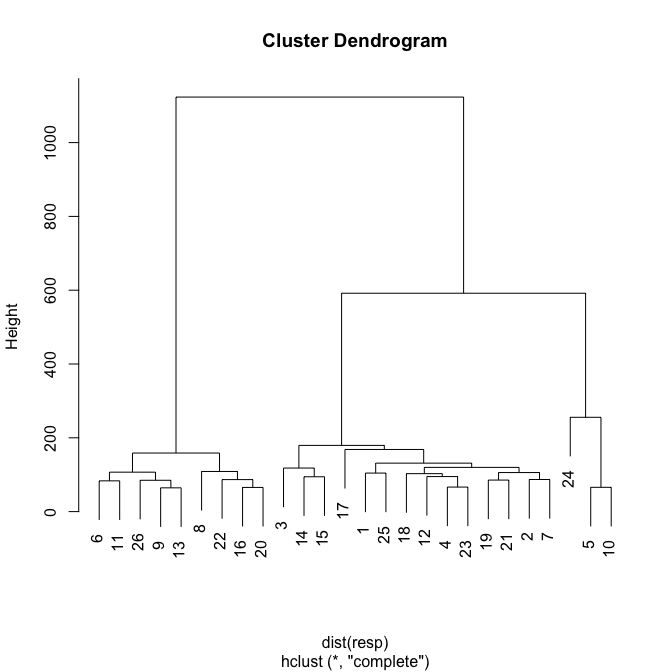The cluster dendrogram clearly indicates there are only three clusters. Making the cut at a height of roughly 300 groups the 26 clusters into only three.

cat("clustra vignette run time:\n")
## clustra vignette run time:
print(proc.time() - start_knit)
##    user  system elapsed
## 157.613   3.128 162.716